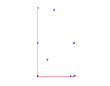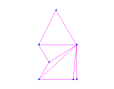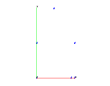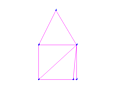Example 1: test using triangulate to create a 2d mesh.

The objective is to use the triangulate command to create a mesh on a polygonal point distribution.

The output consists of four gmv files, two showing the original point distributions, two showing the respective triangulations.

lagrit_input_tri

Images of GMV input and output

Concave point distributiondConcave triangulationConvex point distributionConvex triangulationd